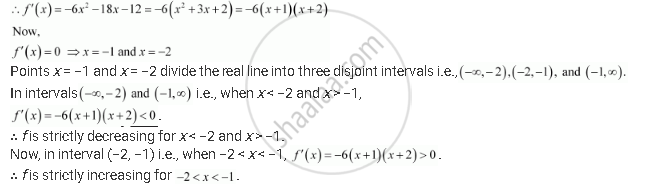# Find the Intervals in Which the Following Functions Are Strictly Increasing Or Decreasing: −2x3 − 9x2 − 12x + 1 - Mathematics

#### Question

Find the intervals in which the following functions are strictly increasing or decreasing:

−2x3 − 9x2 − 12x + 1

#### Solution

We have,

f(x) = −2x3 − 9x2 − 12x + 1Is there an error in this question or solution?

#### APPEARS IN

NCERT Solution for Mathematics Textbook for Class 12 (2018 (Latest))
Chapter 6: Application of Derivatives
| 6.3 | Page no. 205

#### Video TutorialsVIEW ALL 

Find the Intervals in Which the Following Functions Are Strictly Increasing Or Decreasing: −2x3 − 9x2 − 12x + 1 Concept: Increasing and Decreasing Functions.